# Summarizing of Practical Spss Report Case Study

Pages: 195

Words: 48835

## Summarizing of Practical Spss Report Case Study Assignment

Get free samples written by our Top-Notch subject experts for taking Assignment help.

Abstract: Study 1

This particular study will be based on different activities, which will be analyzed in the form of SPSS. However, a procedure of SPSS needs a proper question to be analyzed. Hence, this particular study will analyze the time of meal preparation. In accordance with this, two major variables would be selected, such as Easier to Read and Harder to Read. With the context of these two variables, different data will be given that would be analyzed and interpreted significantly in this study. In the second activity, the descriptive analysis will be performed, and the interpreted data will be discussed in the below section. On the other hand, the third activity is related to the T-Test. It means that both descriptive and T-Test analysis is based on the same question of meal preparation. However, these two analyses will be based on the earlier stated variables as well. The interpretation of data and information of both studies will be based on two variables.

Abstract: Study 2

This particular study would be based on a category between two variables such as the anchor (two-digit number of a mobile phone) and a bid made which is related to a keyboard. These two specific variables will be significantly analyzed in the SPSS, which is done in Study 2. Apart from this, different types of tests will be performed to find out proper relevancy between the two variables. In addition to this, activity two will be performing Calculating Descriptive Statistics. In this test, the researcher will analyze the descriptive analysis between a bid made and an anchor. Similarly, activity three will consist of another test, which is known as Scatter Plot. The consideration of scatter plot will be undertaken because scatterplot is the main analysis, which has the potential to display the major extent related to the correlation or between different values. Apart from this, the last activity will be focused on correlation analysis. Hence, every activity will be based on data and interpretation that will be significantly observed in this study.

### Activity 2: Calculating the Descriptive statistics

After creating and importing the data into the SPSS data file, this file is ready to analyze the output result of the experiment, where the experiment took place in the area of time taking elements for preparing the meal (Li and Deng, 2019). The Mean length of time that participants thought the meal would take to prepare in the Arial condition and in the Brush condition, where the descriptive statistics will be analyzed here.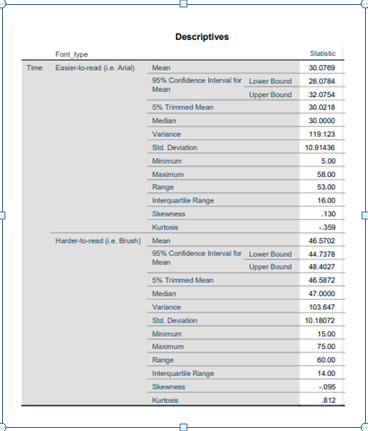According to the above statistics, descriptive is all about the Mean length of time that participants thought the meal would take to prepare in the Arial condition and in the Brush condition, where there are two main elements as Arial condition and the brush condition. Here the Arial condition is seen as easier to read from the time perspective, whereas the brush condition is seen as harder to read from the time perspective. In this above statistics table of descriptive data for Arial, the mean value is 30.0769, where the lower bound for 95% confidence interval for mean is 28.0784, and the upper bound for 95% confidence interval for mean is 32.0754 (Sarikaya and Gleicher, 2017). In this statistics table, the 5% of the trimmed man value is 30.0218, where the value of the median is 30.0000, and the value of the variance is 119.123. In the above table of statistics, the standard deviation value is 10.91436, where the minimum value is 5.00, and the maximum value is 58.00, along with the range of 53.00. The above table of statistics says the value of interquartile is 16.00, where the value of skewness is .130, and the value of kurtosis is -.359. In the above statistics table of descriptive data for Brush, the mean value is 46.5702, where the lower bound for 95% confidence interval for mean is 44.7378, and the upper bound for 95% confidence interval for mean is 48.4027. In this statistics table, the 5% of the trimmed man value is 46.5872, where the value of the median is 47.0000, and the value of the variance is 103.647. In the above table of statistics, the standard deviation value is 10.18072, where the minimum value is 15.00, and the maximum value is 75.00, along with the range of 60.00 (Loeb et al. 2017). The above table of statistics says the value of interquartile is 14.00, where the value of skewness is -.095, and the value of kurtosis is .812.

After discussion of the above statistics, it can be said that there are differences between mean ratings among those groups of the Arial group and the brush group. No, the scores are not similar around those means in those two groups. This data seems to support my hypothesis that a harder to read font will bias people's estimation of how long the meal will take to prepare.

## Activity 3: Conducting an independent sample test

In this section, we are going to discuss and demonstrate the independent sample t-test, where this test will allow us to compare the mean time estimation along with the participants in Bush conditions and Arial condition. There will also be a discussion of different questions and answers such as What is the t-statistic?, What are the degrees of freedom?, Was the p-value less than or greater than 0.05?, Was the difference in time estimates between the Arial and Brush group statistically significant?, Which group had the higher time estimate?, Does this support our hypothesis? and others (Ferraro and Guzmán, 2018). This overall study will help us to understand the SPSS process along with eth descriptive stats and independent T-tests.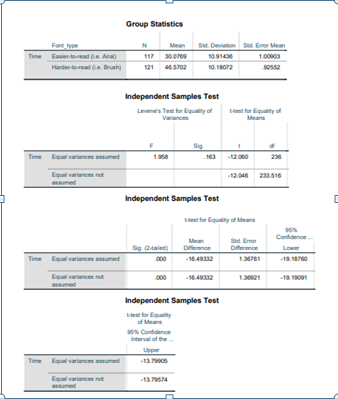The above statistics of independent t-test analysis will be discussed in this section, where the main analysis will be based on the two different groups such as Arial patients group and Bush participants group. Here the Arial condition is seen as easier to read from the time perspective, whereas the brush condition is seen as harder to read from the time perspective. As per the statistical data, the time estimation for Aerial participants is Arial and the Harder to read participants is Bush, where the number of Aerial participants is 117, and the number for Bush participants is 121 (Amrhein et al. 2018). The mean value of the aerial participants is 30.0789, and the mean value of bush participants is 46.5702, along with the standard deviation value for aerial participants is 10.91436, and the standard deviation value for the bush participants is 10.1807. This also talks about the standard error mean value, where the standard error mean value for aerial participants is 1.0903, and the standard error mean value for bush participants is .92552.

1. What is the t-statistic?

T statistics' ' refers to the test of inferential statistics that are mainly used for determining the major difference between the two means groups, where it is related to the features (Zou et al., 2019). It contains degrees of freedom and T statistics for the statistical significance of the hypothesis.

1. What are the degrees of freedom?

Here the degrees of freedom are Aerial participants and Bush participants.

1. Was the p-value less than or greater than 0.05?

Here the p-value was greater than 0.05.

1. Was the difference in time estimates between the Arial and Brush group statistically significant?

Yes, the difference in time estimates between the Arial and Brush group is statistically significant.

1. Which group had the higher time estimate?

This data seems to support my hypothesis that a harder to read font will bias people's estimation.

1. Does this support our hypothesis?

Yes, it supports our hypothesis.

# Practical report of Study 2

## Activity 2: Calculating Descriptive statistics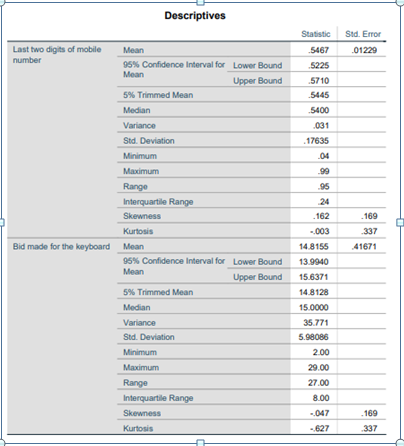After creating and importing the data into the SPSS data file, this file is ready to analyze the output result of the experiment, where the experiment took place in the area of two variables, such as the anchor and the bid made for the keyboard. According to the above statistics, descriptive is all about the two variables such as the anchor and the bid made for the keyboard, where there are two main elements such as two variables such as the anchor and the bid made for the keyboard. Here the anchor is seen as the last two digits of mobile numbers, whereas the bid made for the keyboard is seen as the same.

In this above statistics table of descriptive data for last two digits of mobile numbers, the mean value is .5467, along with the standard error value of .01299, where the lower bound for 95% confidence interval for mean is .5225, and upper bound for 95% confidence interval for mean is .5710. In this statistics table, the 5% of the trimmed man value is .5445, where the value of the median is .5400, and the value of the variance is .031 (Derrick et al. 2017). In the above table of statistics, the standard deviation value is .17635, where the minimum value is .04, and the maximum value is .99, along with the range of .95. The above table of statistics says the value of interquartile is .24, where the value of skewness is .1682 and the value of kurtosis is -.003. Here the standard error for skewness value is .169, and the standard error for kurtosis value is -.337. In this above statistics table of descriptive data for bid made for the keyboard, the mean value is 14.8155, along with the standard error value of .41671, where the lower bound for 95% confidence interval for mean is 13.9940, and upper bound for 95% confidence interval for mean is 15.6371. In this statistics table, the 5% of the trimmed man value is 14.8128, where the value of the median is 15.0000, and the value of the variance is 35.771. In the above table of statistics, the standard deviation value is 5.98086, where the minimum value is 2.00, and the maximum value is 29.00, along with the range of 27.00. The above table of statistics says the value of interquartile is 8.00, where the value of skewness is -.047, and the value of kurtosis is -.627. Here the standard error for skewness value is .169, and the standard error for kurtosis value is -.337 (Chirico et al. 2019). After discussion of the above statistics, it can be said that there are differences between mean ratings among those groups of the last two digits of mobile numbers and the bid made for the keyboard.

## Activity 3: Drawing a scatter plot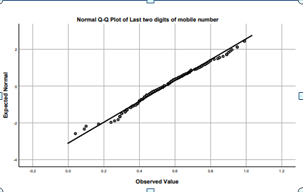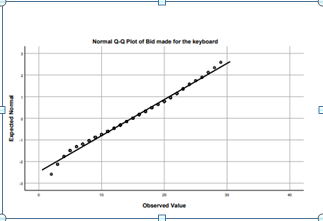In this section, the primary focus point will be on the different scatter plot drawings that will help us to understand where there is a relation between the two different variables or not. Here the anchor is last two digits of mobile numbers, and the bid is the bid made for the keyboard. After analyzing these two scatter plots, it is visible that there is a lack of a strong relationship between the two factors. As in these two different scatter, the plot says that they are in one line and they are closely connected to each other points, where the two different elements are not related much with each other (Anselin, 2019). In simple words, it can be said that the anchor as last two digits of mobile numbers and the bid as the bid made for the keyboard are not connected to each other, or there is no strong relationship between these two factors.

## Activity 4: Calculating your correlation coefficient

In this section, the primary focus point will be on the relation between the two different variables, where the anchor is last two digits of mobile numbers, and the bid is the bid made for the keyboard.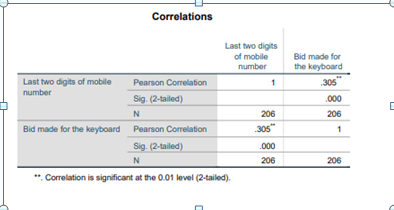In the above statistics of correlation efficiency, there are two main variables such as last two digits of mobile numbers, and the bid made for the keyboard, where the last two digits of mobile numbers'' is the anchor, and bid made for the keyboard is the bid. According to this statistical data the Pearson correlation analysis is used, where the correlation value for last two digits of mobile numbers is 1, and the correlation value for the bid made for the keyboard is .305. After a discussion of the above statistics table, it can be said that there is a lack of a strong relationship between the two factors (Du, 2019). As shown in these two different scatter plots, they are in one line and are tightly connected to each other points, despite the fact that the two different elements are not directly related. In basic terms, the anchor, which is defined as the final two digits of a mobile phone number, and the bid, which is defined as the bid placed for the keyboard, are not connected to one other, or there is no significant tie between them.

# Reference list

Journal

Amrhein, V., Trafimow, D. and Greenland, S., 2018. Inferential statistics as descriptive statistics: there is no replication crisis if we don't expect replication. PeerJ Preprints6, p.e26857v4.

Anselin, L., 2019. The Moran scatterplot as an ESDA tool to assess local instability in spatial association (pp. 111-126). Routledge.

Chirico, F., Heponiemi, T., Pavlova, M., Zaffina, S. and Magnavita, N., 2019. Psychosocial risk prevention in a global occupational health perspective. A descriptive analysis. International journal of environmental research and public health16(14), p.2470.

Derrick, B., Russ, B., Toher, D. and White, P., 2017. Test statistics for the comparison of means for two samples that include both paired and independent observations. Journal of Modern Applied Statistical Methods16(1), p.9.

Du, W.S., 2019. Correlation and correlation coefficient of generalized orthopair fuzzy sets. International Journal of Intelligent Systems34(4), pp.564-583.

Ferraro, R. and Guzmán, M.J., 2018. Quest for the extra degree of freedom in f (T) gravity. Physical Review D98(12), p.124037.

Foley, B., Degirmenci, K. and Yigitcanlar, T., 2020. Factors affecting electric vehicle uptake: Insights from a descriptive analysis in Australia. Urban Science4(4), p.57.

Li, D. and Deng, Y., 2019. A new correlation coefficient based on generalized information quality. IEEE Access7, pp.175411-175419.

Loeb, S., Dynarski, S., McFarland, D., Morris, P., Reardon, S. and Reber, S., 2017. Descriptive Analysis in Education: A Guide for Researchers. NCEE 2017-4023. National Center for Education Evaluation and Regional Assistance.

Loeb, S., Dynarski, S., McFarland, D., Morris, P., Reardon, S. and Reber, S., 2017. Descriptive Analysis in Education: A Guide for Researchers. NCEE 2017-4023. National Center for Education Evaluation and Regional Assistance.

Sarikaya, A. and Gleicher, M., 2017. Scatterplots: Tasks, data, and designs. IEEE transactions on visualization and computer graphics24(1), pp.402-412.

Zou, Q., Ding, L., Wang, H., Wang, J. and Zhang, L., 2019. Two-degree-of-freedom flow-induced vibration of a rotating circular cylinder. Ocean Engineering191, p.106505.

Get your doubts & queries resolved anytime, anywhere.

• On-Time Delivery

• 100% Plagiarism-Free Work

Get original assignments written from scratch.

• UK Expert Writer

Highly-qualified writers with unmatched writing skills.

16000+ Project Delivered
Get best price for your work

Ph.D. Writers For Best Assistance

Plagiarism Free

offer valid for limited time only*